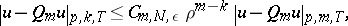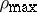# Bramble-Hilbert lemma

Jump to: navigation, search

Hilbert–Bramble lemma

An abstract theoretical tool for studying the approximation error of functions in Sobolev spaces (cf. also Approximation of functions; Sobolev space) by algebraic polynomials. The general formulation of the lemma was given and proven first by J.H. Bramble and S.R. Hilbert [a1] in terms of a class of linear functionals on Sobolev spaces that annihilate the set of polynomials $P _ { K }$ (see the definition below), an intermediate to $P _ { k - 1}$ (polynomials of degree $k - 1$) and $P _ { k }$, i.e. $P _ { k - 1 } \subset P _ { K } \subset P _ { k }$. This formulation was motivated by the work of G. Birkhoff, M. Schultz and R.S. Varga [a3] and applied to estimate the error of the Hermite interpolation formula [a1], spline interpolation and Fourier transformation (cf. Fourier transform) [a2].

The Bramble–Hilbert lemma has a wide range of applications. It is used in the analysis of projection operators in the function spaces $L_{2}$ and $W _ { 2 } ^ { 1 }$ for designing optimal domain decomposition and multi-level methods. Its application to the $L_{2}$-error of the finite element approximations of elliptic problems of second order leads to sharp estimates with respect to both the regularity of the solution and rate of convergence. It is an indispensable tool in the error analysis of finite element [a4], [a5], finite volume [a8], finite difference [a9], collocation, and boundary element methods for solving partial differential equations. The complete error analysis of the finite difference and finite volume schemes in [a9] is based on the Bramble–Hilbert lemma.

To formulate the lemma, some notation regarding Sobolev spaces of real-valued functions on a bounded domain $T$ in $N$-dimensional Euclidean space $\mathbf{R} ^ { N }$ is needed. It is assumed that $T$ satisfies the strong cone condition (cf. Cone condition) and has diameter $\rho = \rho ( T ) = \operatorname { diam } ( T )$. The boundary of $T$ is denoted by $\partial T$.

The notations $\alpha$, $\beta$ and $\gamma$ will be used for multi-indices, with $| \alpha | = \sum _ { j = 1 } ^ { N } \alpha _ { j }$ and $D ^ { \alpha } = D _ { 1 } ^ { \alpha _ { 1 } } \ldots D _ { N } ^ { \alpha _ { N } }$, where $D _ { j } = \partial / \partial x_ { j }$. Let $L _ { p } ( T )$ be the set of all functions $u$ such that $\int _ { T } | u ( x ) | ^ { p } d x$ exists and is finite. The norm in $L _ { p }$ is given by $\| u \| _ { p , T } = ( \int _ { T } | u ( x ) | ^ { p } d x ) ^ { 1 / p }$. Let $W _ { p } ^ { m } ( T )$ be the set of all functions in $L _ { p } ( T )$ whose distributional derivatives of order less than equal to $m$ (a non-negative integer) are in $L _ { p } ( T )$. It will be assumed that $1 \leq p < \infty$. The norm and the semi-norm on $W _ { p } ^ { m } ( T )$ are, respectively,

\begin{equation*} \| u \| _ { p , m , T } = \sum _ { | \alpha | \leq m } \| D ^ { \alpha } u \| _ { p , T }, \end{equation*}

\begin{equation*} | u | _ { p , m , T } = \sum _ { | \alpha | = m } \| D ^ { \alpha } u \| _ { p , T }. \end{equation*}

Let $K$ be any subset of the set of multi-indices $\gamma$ of length $m$ (i.e. $| \gamma | = m$) which contains the indices of the form $\gamma _ { l } = m$, $\gamma_j = 0$ for $j \neq l$, $l = 1 , \ldots , N$. The set of polynomials $q$ such that $D ^ { \gamma } q = 0$ for all $\gamma \in K$ will be denoted by $P _ { K }$. Obviously, $P _ { k - 1 } \subset P _ { K } \subset P _ { k }$.

The Bramble–Hilbert lemma ([a1]) is as follows: Let $F ( u )$ be a bounded linear functional on $W _ { p } ^ { m } ( T )$ that vanishes for polynomials in $P _ { K }$, i.e.

1) $| F ( u ) | \leq C \sum _ { j = 0 } ^ { m } \rho ^ { j - N / p } | u | _ { p , j , T }$, where the constant $C$ is independent of $\rho$ and the function $u$;

2) $F ( q ) = 0$ for $q \in P _ { K }$. Then there is a constant $C _ { 1 }$, independent of $\rho$ and $u$, such that

\begin{equation*} | F ( u ) | \leq C _ { 1 } \sum _ { \alpha \in K } \rho ^ { m - N / p } \| D ^ { \alpha } u \| _ { p , T }. \end{equation*}

Note that the lemma deals with function spaces of many variables. For functions in a single variable, the inequality derived by the lemma follows immediately from the Peano kernel theorem for the remainder of the Taylor expansion (see, e.g., [a10]).

In many applications, a given domain $\Omega$ is split into a number of non-overlapping subdomains $T$, usually triangles, rectangles, tetrahedra, hexahedra, etc., so that $\rho = \rho ( T )$ is small. Consider the error of the Lagrange interpolation formula for a function $u \in W _ { p } ^ { m } ( \Omega )$ with polynomials of degree $m - 1$ over each $T$ and take $\overline { \Omega } = \cup \overline{T}$. Here, the domains $T$ are non-degenerate simplices in $\mathbf{R} ^ { N }$, $\rho = \operatorname { max } _ { T } \rho ( T )$, $K = \{ \gamma : | \gamma | = m \}$, and $P _ { K } = P _ { m - 1 }$. Assume that $p > N$ and $m = 2$ and let $q_{l}$ be a piecewise polynomial of degree $1$ which interpolates the values of the function $u$ at the vertices of each simplex $T$. Consider the linear functional $F ( u ) = u ( x ) - q _{I} ( x )$ at a fixed point $x \in T$. By the Sobolev imbedding theorem (cf. also Imbedding theorems),

\begin{equation*} | u ( x ) | \leq C \sum _ { j = 0 } ^ { 2 } \rho ^ { j - N / p } | u | _ { p , j , T }. \end{equation*}

Therefore $F ( u )$ is bounded in $W _ { p } ^ { m } ( T )$. Obviously, $F ( u ) = 0$ if $u$ is a polynomial of degree $1$, since the linear interpolant recovers $u$ exactly. Therefore, by the Bramble–Hilbert lemma: $| F ( u ) | \leq C _ { 1 } \rho ^ { 2 - N / p } | u | _ { p , 2 , T }$. An estimate of the $L _ { p }$-error for the interpolant $q_{l}$ over $T$ follows immediately after taking this inequality to power $p$ and integrating it over $T$. The estimate for the error in the whole domain $\Omega$ follows by summing all local estimates, taking the maximal $\rho$, and using the additivity of the integrals:

\begin{equation*} \| u - q _ { l } \| _ { p , \Omega } \leq C \rho ^ { 2 } | u | _ { p , 2 , \Omega }. \end{equation*}

If $\rho$ tends to $0$, this estimate gives the rate at which the piecewise-linear interpolant converges to $u$ in the $L _ { p }$-norm. Similarly, one can obtain bounds for the $L _ { p }$-norm of the first derivatives. This is a typical application of the Bramble–Hilbert lemma in constructive function theory and in numerical methods for differential equations.

The original proof of the lemma was abstract and the size of the constant $C _ { 1 }$ could not be estimated. This was the reason that T. Dupont and L.R. Scott [a7] gave a new constructive proof of the main result.

Consider the polynomial $Q_m u$ which is an averaged Taylor polynomial of degree $m - 1$ over $T$ (for the exact construction, see [a4], Chap. 4). This construction of $Q_m u$ is related to the polynomials used by S.L. Sobolev [a11] to study the spaces that bear his name. Here, $T$ is considered to be a star-shaped domain with respect to a ball $B$, that is, for all $x \in B$, the closed convex hull of $\{ x \} \cup B$ is a subset of $T$, and one defines

\begin{equation*} \rho _ { \operatorname { max } } = \operatorname { sup } \{ \rho = \rho ( B ) : T\, \text { star shaped w.r.t. } B \}. \end{equation*}

The revised Bramble–Hilbert lemma now reads ([a4], p. 100): Let $T$ be a star-shaped domain with respect to the ball $B$. Then\begin{equation*} k = 0 , \dots , m. \end{equation*}

Here,is the quotient of $\rho$ and the diameter, and the constant $C _ { m , N, \epsilon}$ does not depend on $\rho$ while its dependence on $m$ and $k$ is estimated explicitly from above.

This variant of the lemma was proved by Dupont and Scott [a7] for $m$ non-integer and for domains that are finite unions of domains that are star-shaped with respect to a ball, while more general results were established in [a6].

How to Cite This Entry:
Bramble-Hilbert lemma. Encyclopedia of Mathematics. URL: http://encyclopediaofmath.org/index.php?title=Bramble-Hilbert_lemma&oldid=50940
This article was adapted from an original article by Raytcho D. Lazarov (originator), which appeared in Encyclopedia of Mathematics - ISBN 1402006098. See original article